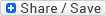Wednesday, March 28, 2007 Will an infection become an epidemic? A simple model   posted by p-ter @ 3/28/2007 03:17:00 PMOne subject I've developed a mild interest in is the modeling of epidemics. So in that vein, here is a post (the first of a possible series, if I feel really inspired) on mathematical epidemiology:Let's start with a simple discrete-time model for the evolution of an infection: imagine an infinite population of people, of which one has become infected with a disease. This one person will infect each of the other susceptible members of the population with some probability p. In the next time unit, the infected individual will recover and become immune to further infection (i.e. he either has developed resistance to the disease or is dead). Each of the newly infected individuals then independently can infect each of the remaining susceptible individuals with probability p once more (so note that the probability of being infected is p if there is one infected individual in the population, 2p if there are two, 3p if there are three, etc), and so on and so forth. The basic model is shown in the figure-- asusceptible individual (S) can be infected, becoming an infected individual (I). Infected individuals then always recover and are removed from the population (R).One of the first (and most mathematically tractable) questions to ask of this model is: will an infection become an epidemic?To answer this question, let's think of the first stages of the infection of the population as a Galton-Watson process (see this wikipedia link if you're wondering what role Francis Galton played in outlining this process). That is, each infected individual infects a random number of susceptible individuals in the next time point according to some probability distribution (called the "offspring distribution") X. If the probability of infecting each individual person is sufficiently low, we can say this distribution is Poisson(lambda). The quantity of interest, then, is the probability that this Galton-Watson process goes extinct; that is, that there exists a time point in which zero people are infected. Let's call this probability E. At a given point in time, if there is one infected individual, the probablity of excinction is E. If there are two infected individuals, the probability of extinction is E^2. Three infected individuals: E^3. And so on. Given that we start with one infected individual, we can than write:E = P(X=0) + P(X=1)E + P(X=2)E^2 + P(X=3)E^3 + P(X=4)E^4 ...Mathematically oriented readers will notice that the right-hand side of this equation is the probability generating function of the offspring distribution, evaluated at E. Indeed, the extinction probability of a Galton-Watson process is the minumum solution to the equation E = A(E) . For our Poisson distribution, this has simple consequences: if the mean of the offspring distribution is less than 1, the infection will not become an epidemic (the only solution to the equation is E = 1). If the mean of the distribution is greater than or equal to one, there is some probability that the Galton-Watson process will never die (in a real population, of course, the epidemic will eventually run out of gas, but we're not interested in those dynamics here). This may seem rather intuitive (if on average, each infected individual infected less than one individual, the infection will die out quickly), but the mathematical formalism allows one to construct more complex models. Plus, reducing lambda (the mean of the Poission distribution), of course, is then the goal of nearly all anti-epidemic precautions-- quarantines, hand-washing, etc.; all can be though of as practical ways to reduce a mathematical parameter.  The probability generation function A(z) of a distribution X is the sum over all possible values of X of P(X=x)z^x. Labels: Epidemiology input{width:180px; border: thin #000000 inset;} .sub{width:180px; border: thin #000000 inset;}Razib's Home Page GNXP Archives Interviews Blogroll